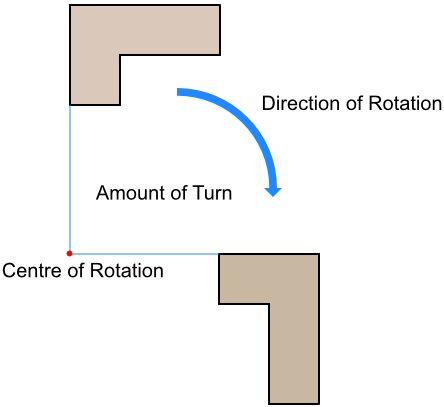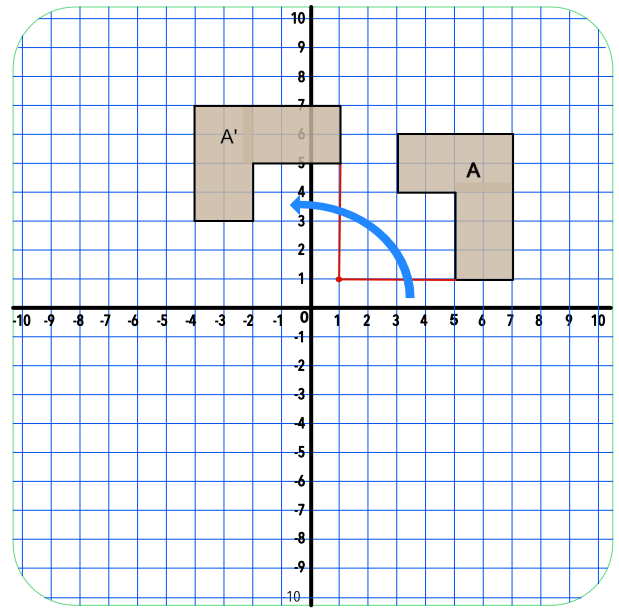Rotation

# Rotation

GCSE(F), GCSE(H),

There are a number of Transformations that can change the position and size of a shape.

A shape is rotated by turning it. To describe a Rotation, the following information is required:

the Centre of Rotation; the shape is turned around that point;

the amount of turn, which might be in degrees or given as a fraction;

the Direction of Rotation, which will be either Clockwise or Anticlockwise.

A rotation is only fully described if all three pieces of information are given (if a rotation is 180º, or a half-turn, the direction is not required as it does not matter in which direction the shape is rotated). Note that a rotated shape is congruent to the original shape.## Examples

1. Rotate the shape A 90º anticlockwise, with a centre of rotation at (1, 1).Answer:2. Describe fully the transformation that moves shape A onto shape B.Answer: The transformation is a rotation.

It is rotated 180º.

The centre of rotation is (0, 0)

Because the shape is turned 180º, it does not matter whether the shape is rotated clockwise or anticlockwise.